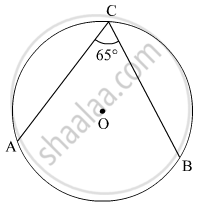# Choose the Correct Alternative: - Geometry

MCQ

Four alternative answers for the following question is given. Choose the correct alternative.

∠ACB is inscribed in arc ACB of a circle with centre O. If ∠ACB = 65°, find m(arc ACB).

• 65°

• 130°

• 295°

• 230°

#### Solution 1The measure of an inscribed angle is half of the measure of the arc intercepted by it.

∴∠ACB = $\frac{1}{2}$ m(arc AB)

⇒ m(arc AB) = 2∠ACB = 2 × 65º = 130º

∴ m(arc ACB) = 360º − m(arc AB) = 360º − 130º = 230º

Hence, the correct answer is 230° .

#### Solution 2

230°

Concept: Inscribed Angle
Is there an error in this question or solution?